### Centers of Equilateral Triangle

Illustration of an equilateral triangle that shows both the centroid (where the medians of the sides…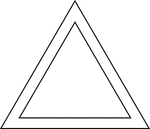### 2 Concentric Equilateral Triangles

Illustration of 2 concentric equilateral triangles.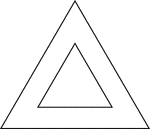### 2 Concentric Equilateral Triangles

Illustration of 2 concentric equilateral triangles.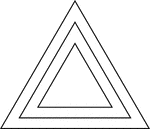### 3 Concentric Equilateral Triangles

Illustration of 3 concentric equilateral triangles that are equally spaced.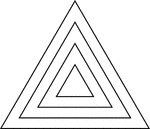### 4 Concentric Equilateral Triangles

Illustration of 4 concentric equilateral triangles that are equally spaced.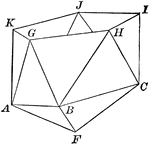### Part of an Icosahedron

Illustration of the bottom part of an icosahedron. The base consists of a regular pyramid, upon which…### Partial Construction of an Icosahedron

Illustration of the steps to a construction of an icosahedron. The base consists of a regular pyramid,…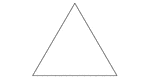### Isosceles Triangle degrees 60, 60, 60

An isosceles triangle with angles 60, 60, 60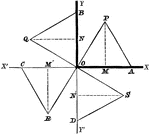### Equilateral Triangles

Equilateral triangles set up along a coordinate plane.